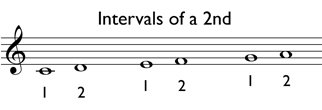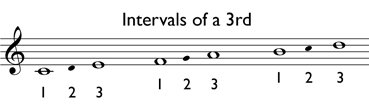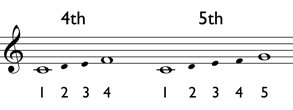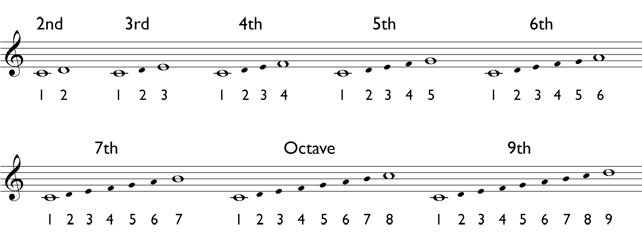# Understanding Numeric Intervals

Step, skip, and leap are general terms that can be used to describe intervals, but they can also be identified more precisely by using numbers. To determine a numeric interval, count the steps between the two pitches. In counting the steps, always include both pitches involved and any staff lines or spaces between them.

For example, the distance between C and D is a 2nd. The distance between E and F is also a 2nd, and so is the distance between G and A. Therefore, the interval of a 2nd is the same as the interval of a step.To determine the interval between C and E, count the pitches as shown below. C is 1, D is 2, and E is 3, indicating that the interval between C and E is a 3rd. F to A is also a 3rd. Therefore, the interval of a 3rd is the same as a skip.The distance from C to F is a 4th, as shown below. The distance from C to G is a 5th. Any interval greater than a 3rd is also called a leap.The example below shows several intervals and how to calculate them. Note that the interval of an 8th is called an octave. When two pitches are the same, the interval is unison.